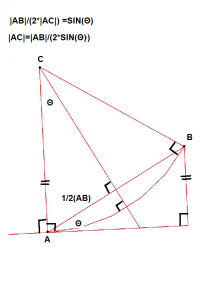So… I’m going to need how to figure out whether or not I need to go cw or ccw when drawing a polyline arc.So what I need to know if the point “B” is basically about the Tangent line to the Arc (ccw ) or below (cw).
Originally my thought was to do a coordinate transformation  to the align the axis to the tangent line and see if B was basically above or below the axis.  This seemed like a alot of work and I asked for input on the freecad IRC

So the suggestion came back:
you have the line (x1,y1), (x2,y2) and you want to connect it to an arc from (x2,y2) to (x3,y3)
I think you just need to calculate the determinant of [x1,x2,x3; y1,y2,y3; 1,1,1]
if it is positive your arc goes in one direction if it is negative it goes in the other direction if it is zero (x3,y3) lies on the line
hmm, not exactly, you can find the right calculation in

 ConstraintP2LDistance::error

you just need to calculate the variable “area” and check its sign

http://people.richland.edu/james/lecture/m116/matrices/applications.html

Ok… All I have to say is this was easy(compared to getting the arcs to draw out correctly)..

switch(Mode){
case STATUS_LINE_SEEK_First:
case STATUS_ARC_SEEK_First:
if (seekAutoConstraint(sugConstr1, onSketchPos, Base::Vector2D(0.f,0.f))) {
renderSuggestConstraintsCursor(sugConstr1);
return;
}
break;
case STATUS_LINE_SEEK_Second:
EditCurve = onSketchPos;
sketchgui->drawEdit(EditCurve);
if (seekAutoConstraint(sugConstr2, onSketchPos, onSketchPos – EditCurve)) {
renderSuggestConstraintsCursor(sugConstr2);
return;
}
break;
case STATUS_ARC_SEEK_Second:
if (getHighestCurveIndex()<0){
//Need to figure if there was a previous element?? In order to find perpendicular to the tangent
//Otherwise there can be infinate solutions do drawing an element.

}
else{
//Need to determine if the previous element was a line or an arc or ???
const Part::Geometry *geom = sketchgui->getSketchObject()->getGeometry(getHighestCurveIndex());
if (geom->getTypeId() == Part::GeomLineSegment::getClassTypeId()) {

const Part::GeomLineSegment *lineSeg = dynamic_cast<const Part::GeomLineSegment *>(geom);
EditCurve = Base::Vector2D(lineSeg->getEndPoint().x, lineSeg->getEndPoint().y);
tangent.Set(lineSeg->getEndPoint().x-lineSeg->getStartPoint().x,
lineSeg->getEndPoint().y-lineSeg->getStartPoint().y,
0.0);

}
else if(geom->getTypeId() == Part::GeomArcOfCircle::getClassTypeId()){

//todo
//need to get some info on the prior arc

}
else{
//It is neither a point or a line.
//todo.. Need to figure out what to do here.

}

//At this point we need to solve for the previous
}

Distance =onSketchPos-EditCurve;
Base::Vector2D tangent2d(tangent.x,tangent.y);

double theta =(double)tangent2d.GetAngle(Distance);

Base::Vector3d kVec;
//At this point we need a unit normal vector pointing torwards then center of the arc we are drawing.
//CCW -1, CW =1
// Derivation of the formula used here can be found here: http://people.richland.edu/james/lecture/m116/matrices/area.html
// Since we can be potentially be dealing with a line or an arc, we need to build the area triangle from the tangent

float x1 = EditCurve.fX;
float y1 = EditCurve.fY;

float x2 = x1+tangent2d.fX;
float y2 = y1+tangent2d.fY;

float x3 = onSketchPos.fX ;
float y3 = onSketchPos.fY;

float twoTimesArea = (x2*y3-x3*y2)-(x1*y3-x3*y1)+(x1*y2-x2*y1);

if (twoTimesArea <0 )
kVec.Set(0.0,0.0,1.0);//CW

else
kVec.Set(0.0,0.0,-1.0);//CCW

Base::Vector3d centerVec = (tangent% kVec);
centerVec.Normalize();